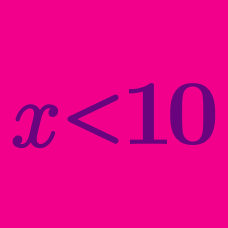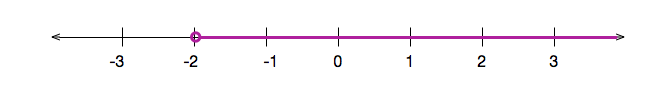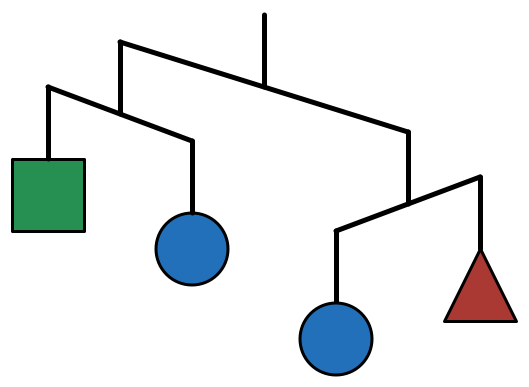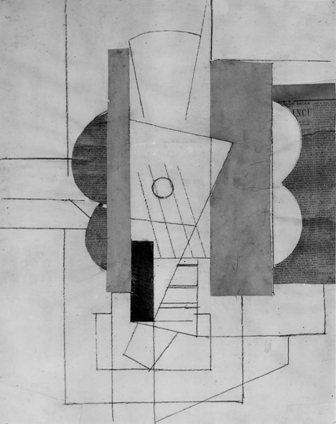Algebra

# Linear Inequalities: Level 1 ChallengesWhich inequality is represented in the above number line?The diagram shows how a mobile will be balanced when left to hang.

What are the relative weights of these shapes?Paula Picasso makes her living by painting. She paints small portraits for $20, large landscapes for$50, and masterpieces for $1000. Paula's goal is to make at least$2800. She has already sold 1 masterpiece and 23 landscapes. How many portraits must she sell to reach her goal?

You should know that

$\frac{a}{b} + \frac{c}{d} \ne \frac{a+c}{b+d}.$

However, if $a, b, c, d$ are positive integers that satisfy $\frac{a}{b} < \frac{c}{d},$ what can we say about $\frac{a+c}{b+d} ?$

These two numbers are very close together:

$\large \frac{122}{353} \approx 0.3456, \ \ \ \ \ \frac{75}{217} \approx 0.3456$

Which of these numbers is larger?

×

Problem Loading...

Note Loading...

Set Loading...